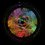# Divisibility by 8

A month or so ago, when I started learning exploring Number Theory, I looked more deeply into divisibility rules. The one that I got from Google for 8 was "A number is divisible by 8 if and only if its last three digits are divisible by 8".

This method is obviously accurate and can be proved really easily. However, it's not that useful... When I see a 3-digit number, I can not tell immediately if it is a multiple of 8. So I thought about how I can verify if 3-digit number is a multiple of 8 very quickly without a calculator, and came up with this.

A number $\overline{...abc}$, (meaning a number ending in the three digits a, b and c) is a multiple of 8 if...

(1) $a$ is even and $\overline{bc}$ is a multiple of 8

OR

(2) $a$ is odd and $\overline{bc}$ is a multiple of 4 and not 8.

This method is much simpler than the one I found from Google, because you need only remember 2-digit multiples of 4 and 8 instead of 3-digit multiples.

I wrote this proof for my method. It's my first attempt at writing a proof, so my format probably won't be very nice, but the mathematics is valid.Note by Nicolas Bryenton
7 years ago

This discussion board is a place to discuss our Daily Challenges and the math and science related to those challenges. Explanations are more than just a solution — they should explain the steps and thinking strategies that you used to obtain the solution. Comments should further the discussion of math and science.

When posting on Brilliant:

• Use the emojis to react to an explanation, whether you're congratulating a job well done , or just really confused .
• Ask specific questions about the challenge or the steps in somebody's explanation. Well-posed questions can add a lot to the discussion, but posting "I don't understand!" doesn't help anyone.
• Try to contribute something new to the discussion, whether it is an extension, generalization or other idea related to the challenge.

MarkdownAppears as
*italics* or _italics_ italics
**bold** or __bold__ bold
- bulleted- list
• bulleted
• list
1. numbered2. list
1. numbered
2. list
Note: you must add a full line of space before and after lists for them to show up correctly
paragraph 1paragraph 2

paragraph 1

paragraph 2

[example link](https://brilliant.org)example link
> This is a quote
This is a quote
    # I indented these lines
# 4 spaces, and now they show
# up as a code block.

print "hello world"
# I indented these lines
# 4 spaces, and now they show
# up as a code block.

print "hello world"
MathAppears as
Remember to wrap math in $$ ... $$ or $ ... $ to ensure proper formatting.
2 \times 3 $2 \times 3$
2^{34} $2^{34}$
a_{i-1} $a_{i-1}$
\frac{2}{3} $\frac{2}{3}$
\sqrt{2} $\sqrt{2}$
\sum_{i=1}^3 $\sum_{i=1}^3$
\sin \theta $\sin \theta$
\boxed{123} $\boxed{123}$